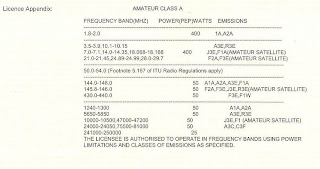## 2009/12/01

### RAE Quiz- 1

A current of 0.5 Ampere flows through a
resistance when 6 Volt is applied. To change the
current to 0.25 Ampere, you must:
a) increased the supply to 12 Volt
b} reduced the supply to 3 Volt
c) connect a 1252 resistor in parallel with ' the existing resistance
d) connect a 6S2 resistor in parallel with the
existing resistor

Answer is B- reduced the supply to 3 Volt

solution:-

Remember the ohm's law formula.

V= IR V is voltage, I is current and R is resistor.

Given I= 0.5A, V= 6v R = ?

V= IR, get R

R= V/R, R= 6v/0.5A
= 12 ohm

if change current to 0.25amp. logically voltage must reduce

given I= 0.25A, v=? and R= 12 ohm

formula V=IR

so V= 0.25A (12 ohm)
= 3v

Check back V=IR

3v= I (12 ohm)
3v/12 ohm = I
so I = 0.25A

The maximum output power permitted from

a Class A amateur radio station is:
a) 300 Watt PEP
b) 400 Watt PEP
c) 500 Watt PEP
d) 1000 Watt PEP

Answer is B- 400 watt pep